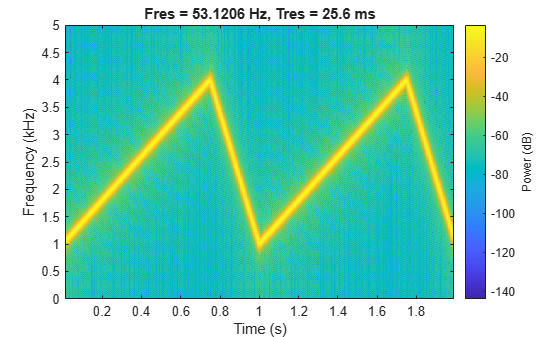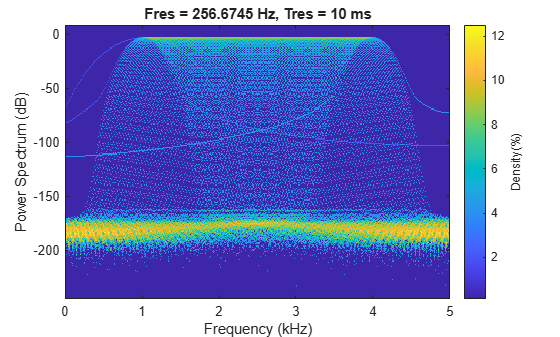# FFT-Based Time-Frequency Analysis

The Signal Processing Toolbox™ product provides functions that return the time-dependent Fourier transform for a sequence, or displays this information as a spectrogram. The time-dependent Fourier transform is the discrete-time Fourier transform for a sequence, computed using a sliding window. This form of the Fourier transform, also known as the short-time Fourier transform (STFT), has numerous applications in speech, sonar, and radar processing. The spectrogram of a sequence is the magnitude of the time-dependent Fourier transform versus time.

To display the spectrogram of a signal, you can use the `pspectrum` function. For example, generate two seconds of a signal sampled at 10 kHz. Specify the instantaneous frequency of the signal as a triangular function of time. To compute the spectrogram, divide the signal into segments of duration 0.0256 second and specify 86% segment-to-segment overlap. The leakage measures the ability of the sliding window to detect a weak tone from noise in the presence of a neighboring strong tone. Specify a leakage of 0.875.

```fs = 10000; t = 0:1/fs:2; x = vco(sawtooth(2*pi*t,0.75),[0.1 0.4]*fs,fs); pspectrum(x,fs,'spectrogram', ... 'TimeResolution',0.0256,'Overlap',86,'Leakage',0.875)```The persistence spectrum of a signal is a time-frequency view that shows the percentage of the time that a given frequency is present in a signal. The persistence spectrum is a histogram in power-frequency space. The longer a particular frequency persists in a signal as the signal evolves, the higher its time percentage and thus the brighter or "hotter" its color in the display.

Compute and display the persistence spectrum of the signal. Specify a time resolution of 0.01 second, 50% overlap between adjoining segments, and a leakage of 0.5.

```pspectrum(x,fs,'persistence', ... 'TimeResolution',0.01,'Overlap',50,'Leakage',0.5)```